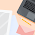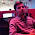Awesome TCS codevita answer for a coding problem

This codevita answer is written in C++ and C programming language.Here is another question which is asked by many people, this is a question asked in codevita. This codevita answer is provided in C++ and C programming language. You can also convert it to java and other programming languages. Lets begin with the coding.

Here is another question which is asked by many people, this is a question asked in codevita. This codevita answer is provided in C++ and C programming language. You can also convert it to java and other programming languages. Lets begin with the coding.

## Codevita Question

Aman and Jasbir are very intelligent guys of their batch. Today they are playing a game "Game of Numbers".

Game Description:

There are N numbers placed on a table. Since two players are playing the game, they will make their moves alternatively. In one move a player can perform the following operation.1. A player will choose a number from the table and will replace that number with one of its divisor. For example, 6 can be replaced with 1, 2, or 3 (since these are the divisors of 6). Similarly, 12 can be replaced with 1, 2, 3, 4 or 6.
2. It is mandatory that the player has to replace the number.
3. A player cannot put back the same number on table.
4. As 1 does not have any divisor other than itself, a player cannot replace 1 with any other number. So soon a situation will arise when there will be only all 1s on the table. In that situation the player will not be able to make any move. The player who will not be able to make the move, loses.
5. Both the players are masters of this game. So they will play the game optimally.
6. Aman will make the first move of the game.
As you will be given N integers that are on the table. You have to predict, who will win the game - Aman or Jasbir.

Input Format:

• First line contains information about the numbers present of the table, denoted by N
• Second line contains N integers delimited by space - A1 A2 A3 A4 -------- AN

Output Format:

• Print the name of the player who will win the game in upper case i.e. AMAN or JASBIR.

Constraints:

• 1 <= N <= 100000
• 0 < Ai <= 10000
• A player cannot replace more than 1 number in one move.
• Players move alternate and a player cannot pass or make no move.
• A number cannot be replaced itself, i.e. 6 can be replaced with 1, 2 or 3 only , not with 6.
• Aman always makes the first move.

## Sample Input and Output for this coding problem

Codevita Sample input and output #1

Input               Output

1                        AMAN

6

Codevita Sample input and output #2

Input               Output

2                       AMAN

18, 6

Codevita Sample input and output #3

Input               Output

4                        JASBIR

24 45 45 24

## The explanation for sample input and output

Codevita sample #1 Explanation:

There is only one number placed on the table do AMAN will replace it with 1. Jasbir will play next. As there is only 1 left on the table. And it cannot be replaced with any other number; he is not able to make any move. So he loses the game.

Also read: TCS codevita old questions for practice

Codevita sample #2 Explanation:

There are 2 numbers present on the table. Since every move is the optimal move, Aman will replace 18 with 6. Now (6, 6) will be left on the table. Now, whatever move Jasbir will make. Aman will also make the same move. So whenever Jasbir will replace any number with 1. In the next move Aman will also replace the other number with 1. So both the numbers will be one after Aman's move. So Jasbir will lose the game.

Initial configuration - (18, 6)
AFTER Aman's MOVE - (6, 6)
AFTER Jasbir's MOVE - (6, 3)
AFTER Aman's MOVE - (3, 3)
AFTER Jasbir's MOVE - (1, 3)
AFTER Aman's MOVE - (1, 1)

Now, Jasbir will not be able to make any move. So, Aman will WIN.

Codevita sample #3 Explanation:

In this case, game is already symmetric. Aman has to make the first move. So whatever move Aman will make Jasbir will just repeat the same. So In the Aman will go out of moves and Jasbir will win the game.

Initial configuration - (24, 45, 45, 24)
AFTER Aman's MOVE - (1, 45, 45, 24)
AFTER Jasbir's MOVE - (1, 45, 45, 1)
AFTER Aman's MOVE - (1, 1, 45, 1)
AFTER Jasbir's MOVE - (1, 1, 1, 1)

Let's see if outcome changes if Aman replaces 24 by 6 instead of 1

Initial configuration - (24, 45, 45, 24)
AFTER Aman's MOVE - (6, 45, 45, 24)
AFTER Jasbir's MOVE - (6, 45, 45, 6)
AFTER Aman's MOVE - (6, 5, 45, 6)
AFTER Jasbir's MOVE - (6, 5, 5, 6)
AFTER Aman's MOVE - (3, 5, 5, 6)
AFTER Jasbir's MOVE - (3, 5, 5, 3)
AFTER Aman's MOVE - (1, 5, 5, 3)
AFTER Jasbir's MOVE - (1, 5, 5, 1)
AFTER Aman's MOVE - (1, 1, 5, 1)
AFTER Jasbir's MOVE - (1, 1, 1, 1)

Now, Aman will not be able to make any move. So, Jasbir will WIN. It is easy to see that just by mimicking Aman's moves, Jasbir will win.

## Codevita answer in C++ Programming

# include<iostream>
using namespace std;
int main()
{
int N,i,sum=0;  //N as an int
int arr;
cin>>N;  //Entering the value of N

for(i=0;i<N;i++){
cin>>arr[i];} //Entering the value in array

for(i=0;i<N;i++){
sum=sum^arr[i];  //Logical value
}

if(sum==0)
cout<<"JASBIR";  //Output the Name of winner
else
cout<<"AMAN";

return 0;
}

## Codevita answer in C programming

# include<stdio.h>
int main(void)
{
int N,i,sum=0;  //N as an int
int arr;
scanf("%d",&N);  //Entering the value of N

for(i=0;i<N;i++){
scanf("%d",&arr[i]);} //Entering the value in array

for(i=0;i<N;i++){
sum=sum^arr[i];  //Logical value
}

if(sum==0)
printf("JASBIR"); //Output the winner name
else
printf("AMAN");

return 0;
}

Hope you liked this post. Ask your query in the comment below. If you think it's not correct or have any issues. Ask or tell it in the comment section below the post.BLOGGER: 5
1.3 tests with wrong answer for the code given here

2.Ok, tell me the errors I will fix them. Or if anybody knows the answer post it here.

1.Run these cases
2
7 3

Jasbir should win....but your code says Aman

3.tell me how to run the program without including the header file iostream
as they have mentioned in the code vita rules

4.## Related Articles

Name

ltr
item
MindxMaster: Awesome TCS codevita answer for a coding problem
Awesome TCS codevita answer for a coding problem
This codevita answer is written in C++ and C programming language.Here is another question which is asked by many people, this is a question asked in codevita. This codevita answer is provided in C++ and C programming language. You can also convert it to java and other programming languages. Lets begin with the coding.